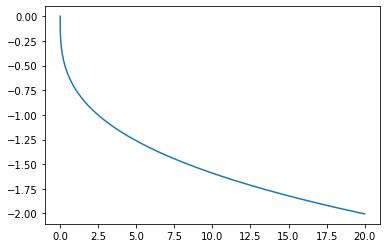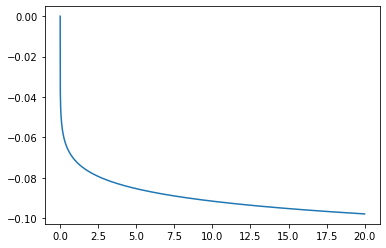6. LDA 泛函核¶

LDA (Local Density Approximation) 的发展可以追溯到 19 世纪 30 年代，它不仅在发展上比 DFT 更早，也同时是 DFT 的基础。同时，LDA 的公式相对来说比较简单，因此程序上可以用较短的代码长度实现。但 LDA 的精度远远不足以处理普遍的化学问题；以后的文档我们会使用 GGA (Generalized Gradient Approximation) 作为基本框架进行计算。因此，这一节我们只是稍稍涉水 DFT 程序；用于以后文档与 pyxdh 的完整的格点积分介绍将会放在下一节的 GGA 自洽场讨论中。

:
import numpy as np
from pyscf import gto, lib, dft
from matplotlib import pyplot as plt
from functools import partial

from pyxdh.Utilities.test_molecules import Mol_H2O2

np.set_printoptions(5, suppress=True, linewidth=120)
np.einsum = partial(np.einsum, optimize=["greedy", 1024 ** 3 * 2 / 8])

DFT 的积分按照约定俗成和一些理论支撑，通常分为交换部分 (Exchange) 与相关部分 (Correlation)。对于 LDA，交换部分是 Slater 泛函 (参考 Wikipedia 页面)；相关部分没有确定的形式，一般使用 VWN5 泛函 [VWN80] (Gaussian 中可能会默认使用 VWN3 泛函，因此使用与 VWN 有关泛函时，需要特别注意这个问题)。

:
mol = Mol_H2O2().mol
grids = Mol_H2O2().gen_grids()

:
nao, ngrids = mol.nao, grids.weights.size
nao, ngrids
:
(22, 130776)

6.1. Slater 交换泛函¶

6.1.1. Slater 交换泛函能量结果¶

Slater 交换泛函能量的表达式是

$\begin{equation} E_\mathrm{x}^{\mathrm{Slater}}[\rho] = - \frac{3}{4}\left( \frac{3}{\pi} \right)^{1/3} \int\rho(\boldsymbol{r})^{4/3} \, \mathrm{d} \boldsymbol{r} \end{equation}$

#p HFS/6-31G nosymm Integral(Grid=99590)

:
scf_eng_s = dft.RKS(mol)
scf_eng_s.grids = grids
scf_eng_s.xc = "Slater"
scf_eng_s.kernel()
:
-149.06413916042908

:
config = {"scf_eng": scf_eng_s}

$E_\mathrm{elec}[D_{\mu \nu}] = (h_{\mu \nu} + \frac{1}{2} J_{\mu \nu} [D_{\kappa \lambda}]) D_{\mu \nu} + E_\mathrm{x}^\mathrm{Slater} [\rho]$

$E_\mathrm{x}^\mathrm{Slater} [\rho] = E_\mathrm{elec}[D_{\mu \nu}] - (h_{\mu \nu} + \frac{1}{2} J_{\mu \nu} [D_{\kappa \lambda}]) D_{\mu \nu}$
:
eng_xc_s = scf_eng_s.energy_elec() - ((scfh_s.H_0_ao + 0.5 * scf_eng_s.get_j()) * scfh_s.D).sum()
eng_xc_s
:
-15.553774010932813

6.1.2. 轨道格点¶

• 下标 $$g$$ 代表 DFT 格点。

• $$w_g$$ 代表 DFT 格点所具有的权重。

$E_\mathrm{x}^{\mathrm{Slater}}[\rho] = - \frac{3}{4}\left( \frac{3}{\pi} \right)^{1/3} w_g \rho_g^{4/3}$

:
ni = dft.numint.NumInt()

:
grid_ao, _, grid_weights, grid_coords = np.zeros((4, ngrids, nao)), _, np.zeros(ngrids), np.zeros((ngrids, 3))
g_start, g_end, g_mem = 0, 0, 2000
for ao, mask, weights, coords in ni.block_loop(mol, grids, nao, 1, g_mem):
g_end = g_start + ao.shape
g_slice = slice(g_start, g_end)
grid_ao[:, g_slice, :] = ao
grid_weights[g_slice] = weights
grid_coords[g_slice] = coords
g_start = g_end

:
print(np.allclose(grid_weights, grids.weights))
print(np.allclose(grid_coords, grids.coords))
True
True

1. 请尽可能解释生成 grid_aogrid_weightsgrid_coords 代码的过程。

2. 下面一行代码似乎能直接生成 grid_aogrid_weightsgrid_coords 三者；但作者不推荐这么使用代码。请解释原因。

grid_ao, _, grid_weights, grid_coords = next(ni.block_loop(mol, grids, nao, 1, g_mem))

提示：首先注意到 ni.block_loop 的返回量是迭代器，而 next 在 Python 中是用来返回迭代器中下一个迭代量；其次思考或通过 Hacking 程序或查看 API 文档了解 g_mem 参数的意义。

:
ao_0 = grid_ao
ao_0.shape
:
(130776, 22)

6.1.3. 密度格点¶

$\rho (\boldsymbol{r}) = \phi_i (\boldsymbol{r}) \phi_i (\boldsymbol{r})$

$\phi_i (\boldsymbol{r}) = C_{\mu i} \phi_\mu (\boldsymbol{r})$

$\rho (\boldsymbol{r}) = C_{\mu i} C_{\nu i} \phi_\mu (\boldsymbol{r}) \phi_\nu (\boldsymbol{r}) = D_{\mu \nu} \phi_\mu (\boldsymbol{r}) \phi_\nu (\boldsymbol{r})$

$\rho_g = D_{\mu \nu} \phi_{g \mu} \phi_{g \nu}$
:
rho_s_0 = np.einsum("uv, gu, gv -> g", scfh_s.D, ao_0, ao_0)

$n_\mathrm{elec} = \int \rho(\boldsymbol{r}) \, \mathrm{d} \boldsymbol{r}$

$n_\mathrm{elec} = w_g \rho_g$
:
(rho_s_0 * grids.weights).sum()
:
18.00000019228845

1. 我们只说生成了密度格点，但我们其实还没有进行过量纲分析。请根据电子数格点积分的程序结果，判断 rho_s_0 $$\rho_g$$ 向量中每个元素的量纲是什么。

提示：格点权重 $$w_g$$ 的量纲认为是 $$[\mathrm{L}]^3$$

6.1.4. Slater 交换泛函能量¶

$E_\mathrm{x}^{\mathrm{Slater}}[\rho] = - \frac{3}{4}\left( \frac{3}{\pi} \right)^{1/3} \int \rho(\boldsymbol{r})^{4/3} \, \mathrm{d} \boldsymbol{r}$

$E_\mathrm{x}^{\mathrm{Slater}}[\rho] = - \frac{3}{4}\left( \frac{3}{\pi} \right)^{1/3} w_g \rho_g^{4/3}$
:
(- 3/4 * (3/np.pi)**(1/3) * rho_s_0**(4/3) * grids.weights).sum()
:
-15.553774010932845

6.1.5. Slater 交换泛函核¶

Slater 交换泛函由于形式非常简单，可以直接给出交换相关泛函的能量；但一般来说，泛函是通过核 (kernel) $$f[\rho]$$ 先定义，随后根据下式给出泛函能量：

$E_\mathrm{x}^{\mathrm{Slater}}[\rho] = \int f^\mathrm{Slater} [\rho] \rho(\boldsymbol{r}) \, \mathrm{d} \boldsymbol{r}$

$f^\mathrm{Slater} [\rho] = - \frac{3}{4}\left( \frac{3}{\pi} \right)^{1/3} \rho^{1/3}$

$f_g = - \frac{3}{4}\left( \frac{3}{\pi} \right)^{1/3} \rho^{1/3}_g$
:
kernel_s = - 3/4 * (3/np.pi)**(1/3) * rho_s_0**(1/3)

$E_\mathrm{x}^{\mathrm{Slater}}[\rho] = w_g f_g \rho_g$
:
(grids.weights * kernel_s * rho_s_0).sum()
:
-15.553774010932846

6.1.6. PySCF 程序计算 Slater 交换能¶

:
exc_s = ni.eval_xc("Slater", rho_s_0, deriv=0)

:
np.allclose(exc_s, kernel_s)
:
False
:
np.allclose(exc_s * rho_s_0, kernel_s * rho_s_0)
:
True

6.1.7. 改编 LibXC 源代码给出泛函核¶

:
# system & method insensitive
Pi = np.pi
RS_FACTOR = (3/(4*Pi))**(1/3)
X_FACTOR_C = 3/8*(3/Pi)**(1/3)*4**(2/3)
# system & method sensitive
z = 0
def rs(rho): return RS_FACTOR * (rho + 1e-50)**(-1/3)

\begin{equation} E_\mathrm{xc}^{X\alpha}[\rho] = - \frac{9}{8} \alpha \left( \frac{3}{\pi} \right)^{1/3}\int\rho(\boldsymbol{r})^{4/3} \, \mathrm{d} \boldsymbol{r} \end{equation}

:
params_a_alpha = 1
lda_x_ax = -params_a_alpha*RS_FACTOR*X_FACTOR_C/2**(4/3)
def ker_lda_x(rs, z):
return lda_x_ax*((1 + z)**(4/3) + (1 - z)**(4/3))/rs

:
np.allclose(ker_lda_x(rs(rho_s_0), 0), kernel_s)
:
True

……以及能给出正确的泛函能量 $$E_\mathrm{x}^\mathrm{Slater} [\rho] = w_g f_g \rho_g$$

:
(grids.weights * ker_lda_x(rs(rho_s_0), 0) * rho_s_0).sum()
:
-15.553774010932845

6.1.8. Slater 交换泛函核的图像¶

:
scaler_rho = np.arange(0, 20, 0.01)
plt.plot(scaler_rho, ker_lda_x(rs(scaler_rho), 0))
:
[<matplotlib.lines.Line2D at 0x7fbb04298b00>]6.2. VWN5 相关泛函¶

VWN5 积分是 LDA 方法中最常用的相关能计算方法。在 Gaussian 中，若打算仅仅计算 VWN5 相关能，输入卡中可以包含下述语句：

#p SVWN5/6-31G nosymm Integral(Grid=99590) IOp(3/74=1)

VWN5 的公式较为复杂，在此我们不打算从公式来给出程序实现；但会给出从 LibXC 代码转换到 Python 代码的过程。首先，我们进行一次 VWN5 的计算过程：

:
scf_eng_vwn = dft.RKS(mol)
scf_eng_vwn.xc = "VWN5"
scf_eng_vwn.grids = grids
scf_eng_vwn.kernel()

config = {"scf_eng": scf_eng_vwn}

:
rho_vwn_0 = np.einsum("uv, gu, gv -> g", scfh_vwn.D, ao_0, ao_0)
exc_vwn = ni.eval_xc("VWN5", rho_vwn_0, deriv=0)

:
eng_xc_vwn = scf_eng_vwn.energy_elec() - ((scfh_vwn.H_0_ao + 0.5 * scf_eng_vwn.get_j()) * scfh_vwn.D).sum()
eng_xc_vwn
:
-1.196157442939949

6.2.1. 根据 LibXC 源代码给出泛函核¶

:
def f_zeta(z): return ((1 + z)**(4/3) + (1 - z)**(4/3) - 2)/(2**(4/3) - 2)

vwn.mpl 中的定义如下 (由于 Maple 是 1-Indexing，因此我们在 Python 代码中的所有 0 索引位用 None 填充)：

:
A_vwn  = [None,  0.0310907, 0.01554535, -1/(6*Pi**2)]
b_vwn  = [None,  3.72744,   7.06042,    1.13107  ]
c_vwn  = [None, 12.9352,   18.0578,    13.0045   ]
x0_vwn = [None, -0.10498,  -0.32500,   -0.0047584]

A_rpa  = [None,  0.0310907,  0.01554535,  -1/(6*Pi**2)]
b_rpa  = [None, 13.0720,    20.1231,      1.06835  ]
c_rpa  = [None, 42.7198,   101.578,      11.4813   ]
x0_rpa = [None, -0.409286,  -0.743294,   -0.228344 ]

def Q(b, c): return np.sqrt(4*c - b**2)
def f1(b, c): return 2*b/Q(b, c)
def f2(b, c, x0): return b*x0/(x0**2 + b*x0 + c)
def f3(b, c, x0): return 2*(2*x0 + b)/Q(b, c)
fpp = 4/(9*(2**(1/3) - 1))

def fx(b, c, rs): return rs + b*np.sqrt(rs) + c

def f_aux(A, b, c, x0, rs): return A*(
+ np.log(rs/fx(b, c, rs))
+ (f1(b, c) - f2(b, c, x0)*f3(b, c, x0))*np.arctan(Q(b, c)/(2*np.sqrt(rs) + b))
- f2(b, c, x0)*np.log((np.sqrt(rs) - x0)**2/fx(b, c, rs))
)

def DMC(rs, z): return(
+ f_aux(A_vwn, b_vwn, c_vwn, x0_vwn, rs)
- f_aux(A_vwn, b_vwn, c_vwn, x0_vwn, rs)
)

:
def ker_lda_c_vwn(rs, z): return(
+ f_aux(A_vwn, b_vwn, c_vwn, x0_vwn, rs)
+ f_aux(A_vwn, b_vwn, c_vwn, x0_vwn, rs)*f_zeta(z)*(1 - z**4)/fpp
+ DMC(rs, z)*f_zeta(z)*z**4
)

:
np.allclose(ker_lda_c_vwn(rs(rho_vwn_0), 0) * rho_vwn_0, exc_vwn * rho_vwn_0)
:
True

LibXC 改编代码给出的相关能 $$E_\mathrm{c}^\mathrm{VWN5} [\rho] = w_g f_g \rho_g$$

:
(grids.weights * ker_lda_c_vwn(rs(rho_vwn_0), 0) * rho_vwn_0).sum()
:
-1.1961574429398458

6.2.2. VWN5 相关泛函核的图像¶

:
scaler_rho = np.arange(0, 20, 0.01)
plt.plot(scaler_rho, ker_lda_c_vwn(rs(scaler_rho), 0))
:
[<matplotlib.lines.Line2D at 0x7fbb041cb390>]6.3. 参考任务解答¶

6.3.1. 任务 (1)¶

6.3.1.1. 任务 (1.1)¶

• Line 1：是为格点张量预留出足够的空间。

• grid_ao 是三维张量，其中第一维度表示求导梯度，当取为 0, 1, 2, 3 时分别代表没有求导、$$\partial_x$$$$\partial_y$$$$\partial_z$$

• 第二维度是格点。

• 第三维度是 AO 基组。在这篇文档中，我们只使用没有求导的部分，即 grid_aoao_0 $$\phi_{g \mu}$$；而被求导的部分则是 grid_ao[1:4] $$\phi_{rg \mu} = \partial_r \phi_{g \mu}$$

• Line 2：指定每次迭代过程的格点起点指标 g_start、终点指标 g_end，以及给予分配内存的大小 g_memg_startg_end 在后续迭代过程中会变更，但 g_mem 是固定值；g_mem = 2000 意指生成轨道格点过程预留 2000 MB。

• Line 3：对 ni.block_loop 返回的迭代器进行迭代。ni.block_loop 传入的第四个参数 1 指的是求轨道的一阶导数 $$\phi_{rg \mu}$$；若传入 3 则意为求三阶导数 $$\phi_{tsrg \mu} = \partial_t \partial_s \partial_r \phi_{g \mu}$$。该值也会对 grid_ao 应当具有的维度有所影响；当传入 1grid_ao 的第一维度应当是 4，而传入 3 时则应当是 $$1 + 3 + 6 + 10 = 20$$

• Line 4-5：根据 ni.block_loop 所给出的格点数量，向 grid_ao, grid_weights, grid_coords 等变量进行填充。

• 后面的代码便是填入张量，并且更新下一次迭代时的格点指标起点为这次迭代时的格点指标终点。

6.3.1.2. 任务 (1.2)¶

:
g_mem = 2000
grid_ao_wrong, _, grid_weights_wrong, grid_coords_wrong = next(ni.block_loop(mol, grids, nao, 1, g_mem))
print(np.allclose(grid_weights_wrong, grids.weights))
print(np.allclose(grid_coords_wrong, grids.coords))
print(grid_ao_wrong.shape)
True
True
(4, 130776, 22)

:
g_mem = 10
grid_ao_wrong, _, grid_weights_wrong, grid_coords_wrong = next(ni.block_loop(mol, grids, nao, 1, g_mem))
print(grid_ao_wrong.shape)
(4, 7040, 22)

DFT 格点由于体积非常庞大，因此对于较大的分子或者交密集的格点，即使计算机的 RAM 再大也可能撑不住一次性在内存读入所有格点，更不提基于这些格点的运算了。所幸的是，对于任何格点积分运算，其中的一个格点的结果不会影响到另一个格点；或者说将 DFT 的总的格点进行分批 (batch) $$g = \{ g_1, g_2, \cdots, g_n \}$$，那么

$\int f(\boldsymbol{r}) \, \mathrm{d} \boldsymbol{r} = \sum_g w_g f_g = \sum_{g} \sum_{i = 1}^{n} w_{g_i} f_{g_i}$

6.3.2. 任务 (2)¶

6.3.2.1. 任务 (2.1)¶

$$\rho_g$$ 在每个格点上的元素的量纲是 $$[\mathrm{L}]^{-3}$$

$\int f(x) \, \mathrm{d} x \sim \sum_g f_g \Delta x$

6.4. 参考文献¶

VWN80

S. H. Vosko, L. Wilk, and M. Nusair. Accurate spin-dependent electron liquid correlation energies for local spin density calculations: a critical analysis. Can. J. Phys., 58(8):1200–1211, aug 1980. doi:10.1139/p80-159.